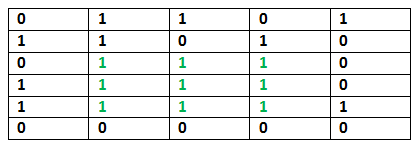# C# Program for Maximum size square sub-matrix with all 1s

Given a binary matrix, find out the maximum size square sub-matrix with all 1s.

For example, consider the below binary matrix.## C#

 `// C# Code for Maximum size square ` `// sub-matrix with all 1s ` ` `  `using` `System; ` ` `  `public` `class` `GFG { ` `    ``// method for Maximum size square sub-matrix with all 1s ` `    ``static` `void` `printMaxSubSquare(``int``[, ] M) ` `    ``{ ` `        ``int` `i, j; ` `        ``// no of rows in M[, ] ` `        ``int` `R = M.GetLength(0); ` `        ``// no of columns in M[, ] ` `        ``int` `C = M.GetLength(1); ` `        ``int``[, ] S = ``new` `int``[R, C]; ` ` `  `        ``int` `max_of_s, max_i, max_j; ` ` `  `        ``/* Set first column of S[, ]*/` `        ``for` `(i = 0; i < R; i++) ` `            ``S[i, 0] = M[i, 0]; ` ` `  `        ``/* Set first row of S[][]*/` `        ``for` `(j = 0; j < C; j++) ` `            ``S[0, j] = M[0, j]; ` ` `  `        ``/* Construct other entries of S[, ]*/` `        ``for` `(i = 1; i < R; i++) { ` `            ``for` `(j = 1; j < C; j++) { ` `                ``if` `(M[i, j] == 1) ` `                    ``S[i, j] = Math.Min(S[i, j - 1], ` `                                       ``Math.Min(S[i - 1, j], S[i - 1, j - 1])) ` `                              ``+ 1; ` `                ``else` `                    ``S[i, j] = 0; ` `            ``} ` `        ``} ` ` `  `        ``/* Find the maximum entry, and indexes of  ` `            ``maximum entry in S[, ] */` `        ``max_of_s = S[0, 0]; ` `        ``max_i = 0; ` `        ``max_j = 0; ` `        ``for` `(i = 0; i < R; i++) { ` `            ``for` `(j = 0; j < C; j++) { ` `                ``if` `(max_of_s < S[i, j]) { ` `                    ``max_of_s = S[i, j]; ` `                    ``max_i = i; ` `                    ``max_j = j; ` `                ``} ` `            ``} ` `        ``} ` ` `  `        ``Console.WriteLine(``"Maximum size sub-matrix is: "``); ` `        ``for` `(i = max_i; i > max_i - max_of_s; i--) { ` `            ``for` `(j = max_j; j > max_j - max_of_s; j--) { ` `                ``Console.Write(M[i, j] + ``" "``); ` `            ``} ` `            ``Console.WriteLine(); ` `        ``} ` `    ``} ` ` `  `    ``// Driver program ` `    ``public` `static` `void` `Main() ` `    ``{ ` `        ``int``[, ] M = ``new` `int``[6, 5] { { 0, 1, 1, 0, 1 }, ` `                                    ``{ 1, 1, 0, 1, 0 }, ` `                                    ``{ 0, 1, 1, 1, 0 }, ` `                                    ``{ 1, 1, 1, 1, 0 }, ` `                                    ``{ 1, 1, 1, 1, 1 }, ` `                                    ``{ 0, 0, 0, 0, 0 } }; ` ` `  `        ``printMaxSubSquare(M); ` `    ``} ` `} `

Output:

```Maximum size sub-matrix is:
1 1 1
1 1 1
1 1 1
```

Please refer complete article on Maximum size square sub-matrix with all 1s for more details!

My Personal Notes arrow_drop_up
Article Tags :

Be the First to upvote.

Please write to us at contribute@geeksforgeeks.org to report any issue with the above content.